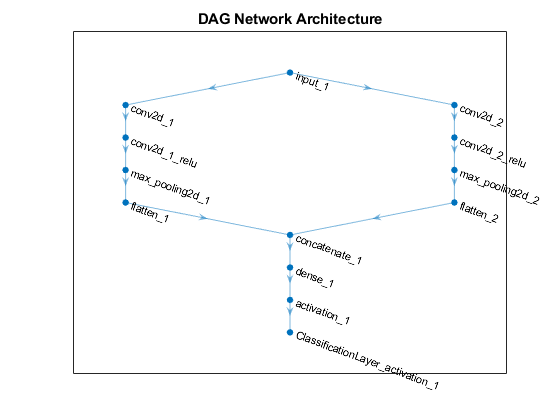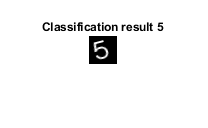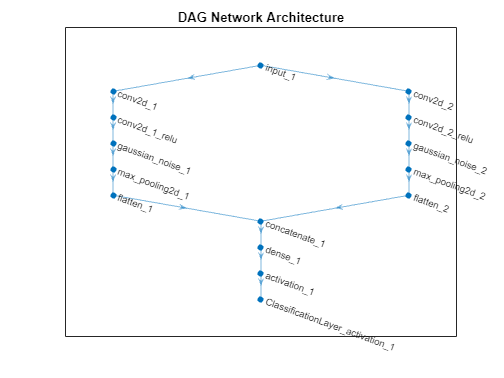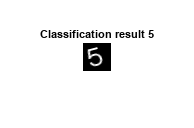importTensorFlowNetwork

Import pretrained TensorFlow network

Description

example

net = importTensorFlowNetwork(modelFolder) imports a pretrained TensorFlow™ network from the folder modelFolder, which contains the model in the saved model format (compatible only with TensorFlow 2). The function can import TensorFlow networks created with the TensorFlow-Keras sequential or functional API. importTensorFlowNetwork imports the layers defined in the saved_model.pb file and the learned weights contained in the variables subfolder, and returns the network net as a DAGNetwork or dlnetwork object.

importTensorFlowNetwork requires the Deep Learning Toolbox™ Converter for TensorFlow Models support package. If this support package is not installed, then importTensorFlowNetwork provides a download link.

Note

importTensorFlowNetwork tries to generate a custom layer when you import a custom TensorFlow layer or when the software cannot convert a TensorFlow layer into an equivalent built-in MATLAB® layer. For a list of layers for which the software supports conversion, see TensorFlow-Keras Layers Supported for Conversion into Built-In MATLAB Layers.

importTensorFlowNetwork saves the generated custom layers and the associated TensorFlow operators in the package +modelFolder.

importTensorFlowNetwork does not automatically generate a custom layer for each TensorFlow layer that is not supported for conversion into a built-in MATLAB layer. For more information on how to handle unsupported layers, see Tips.

example

net = importTensorFlowNetwork(modelFolder,Name,Value) imports the pretrained TensorFlow network with additional options specified by one or more name-value arguments. For example, 'OutputLayerType','classification' imports the network as a DAGNetwork with a classification output layer appended to the end of the imported network architecture.

Examples

collapse all

Import a pretrained TensorFlow network in the saved model format as a DAGNetwork object, and use the imported network to classify an image.

Specify the model folder.

if ~exist('digitsDAGnet','dir')
unzip('digitsDAGnet.zip')
end
modelFolder = './digitsDAGnet';

Specify the class names.

classNames = {'0','1','2','3','4','5','6','7','8','9'};

Import a TensorFlow network in the saved model format. By default, importTensorFlowNetwork imports the network as a DAGNetwork object. Specify the output layer type for an image classification problem.

net = importTensorFlowNetwork(modelFolder,'OutputLayerType','classification','Classes',classNames)
Importing the saved model...
Translating the model, this may take a few minutes...
Finished translation. Assembling network...
Import finished.

net =
DAGNetwork with properties:

Layers: [13×1 nnet.cnn.layer.Layer]
Connections: [13×2 table]
InputNames: {'input_1'}
OutputNames: {'ClassificationLayer_activation_1'}

Plot the network architecture.

plot(net)
title('DAG Network Architecture')Read the image you want to classify and display the size of the image. The image is a grayscale (one-channel) image with size 28-by-28 pixels.

digitDatasetPath = fullfile(toolboxdir('nnet'),'nndemos','nndatasets','DigitDataset');
size(I)
ans = 1×2

28    28

Display the input size of the network. In this case, the image size matches the network input size. If they do not match, you must resize the image by using imresize(I, netInputSize(1:2)).

net.Layers(1).InputSize
ans = 1×3

28    28     1

Classify the image using the pretrained network.

label = classify(net,I);

Display the image and the classification result.

imshow(I)
title(['Classification result ' char(label)])Import a pretrained TensorFlow Network in the saved model format as a dlnetwork object, and use the imported network to predict class labels.

Specify the model folder.

if ~exist('digitsDAGnet','dir')
unzip('digitsDAGnet.zip')
end
modelFolder = './digitsDAGnet';

Specify the class names.

classNames = {'0','1','2','3','4','5','6','7','8','9'};

Import a TensorFlow network in the saved model format as a dlnetwork object.

net = importTensorFlowNetwork(modelFolder,'TargetNetwork','dlnetwork')
Importing the saved model...
Translating the model, this may take a few minutes...
Finished translation. Assembling network...
Import finished.

net =
dlnetwork with properties:

Layers: [12×1 nnet.cnn.layer.Layer]
Connections: [12×2 table]
Learnables: [6×3 table]
State: [0×3 table]
InputNames: {'input_1'}
OutputNames: {'activation_1'}
Initialized: 1

Read the image you want to classify and display the size of the image. The image is a grayscale (one-channel) image with size 28-by-28 pixels.

digitDatasetPath = fullfile(toolboxdir('nnet'),'nndemos','nndatasets','DigitDataset');
size(I)
ans = 1×2

28    28

Display the input size of the network. In this case, the image size matches the network input size. If they do not match, you must resize the image by using imresize(I, netInputSize(1:2)).

netInputSize = net.Layers(1).InputSize
netInputSize = 1×3

28    28     1

Convert the image to a dlarray. Format the images with the dimensions 'SSCB' (spatial, spatial, channel, batch). In this case, the batch size is 1 and you can omit it ('SSC').

I_dlarray = dlarray(single(I),'SSCB');

Classify the sample image and find the predicted label.

prob = predict(net,I_dlarray);
[~,label] = max(prob);

Display the image and the classification result.

imshow(I)
title(['Classification result ' classNames{label}])Import a pretrained TensorFlow network in the saved model format as a DAGNetwork object, and use the imported network to classify an image. The imported network contains layers that are not supported for conversion into built-in MATLAB layers. The software automatically generates custom layers when you import these layers.

This example uses the helper function findCustomLayers. To view the code for this function, see Helper Function.

Specify the model folder.

if ~exist('digitsDAGnetwithnoise','dir')
unzip('digitsDAGnetwithnoise.zip')
end
modelFolder = './digitsDAGnetwithnoise';

Specify the class names.

classNames = {'0','1','2','3','4','5','6','7','8','9'};

Import a TensorFlow network in the saved model format. By default, importTensorFlowNetwork imports the network as a DAGNetwork object. Specify the output layer type for an image classification problem.

net = importTensorFlowNetwork(modelFolder,'OutputLayerType','classification','Classes',classNames);
Importing the saved model...
Translating the model, this may take a few minutes...
Finished translation. Assembling network...
Import finished.

If the imported network contains layers not supported for conversion into built-in MATLAB layers, then importTensorFlowNetwork can automatically generate custom layers in place of these layers. importTensorFlowNetwork saves each generated custom layer to a separate .m file in the package +digitsDAGnetwithnoise in the current folder.

Find the indices of the automatically generated custom layers using the helper function findCustomLayers, and display the custom layers.

ind = findCustomLayers(net.Layers,'+digitsDAGnetwithnoise');
net.Layers(ind)
ans =
2×1 Layer array with layers:

1   'gaussian_noise_1'   GaussianNoise   digitsDAGnetwithnoise.kGaussianNoise1Layer3766
2   'gaussian_noise_2'   GaussianNoise   digitsDAGnetwithnoise.kGaussianNoise2Layer3791

Plot the network architecture.

plot(net)
title('DAG Network Architecture')Read the image you want to classify.

digitDatasetPath = fullfile(toolboxdir('nnet'),'nndemos','nndatasets','DigitDataset');

Classify the image using the pretrained network.

label = classify(net,I);

Display the image and the classification result.

imshow(I)
title(['Classification result ' char(label)])Helper Function

This section provides the code of the helper function findCustomLayers used in this example. findCustomLayers returns the indices of the custom layers that importTensorFlowNetwork automatically generates.

function indices = findCustomLayers(layers,PackageName)

s = what(['.\' PackageName]);

indices = zeros(1,length(s.m));
for i = 1:length(layers)
for j = 1:length(s.m)
if strcmpi(class(layers(i)),[PackageName(2:end) '.' s.m{j}(1:end-2)])
indices(j) = i;
end
end
end

end

Input Arguments

collapse all

Name of the folder containing the TensorFlow model, specified as a character vector or string scalar. modelFolder must be in the current folder, or you must include a full or relative path to the folder. modelFolder must contain the file saved_model.pb, and the subfolder variables. It can also contain the subfolders assets and assets.extra.

• The file saved_model.pb contains the layer graph architecture and training options (for example, optimizer, losses, and metrics).

• The subfolder variables contains the weights learned by the pretrained TensorFlow network. By default, importTensorFlowNetwork imports the weights.

• The subfolder assets contains supplementary files (for example, vocabularies), which the layer graph can use. importTensorFlowNetwork does not import the files in assets.

• The subfolder assets.extra contains supplementary files (for example, information for users), which coexist with the layer graph.

Example: 'MobileNet'

Example: './MobileNet'

Name-Value Arguments

Specify optional comma-separated pairs of Name,Value arguments. Name is the argument name and Value is the corresponding value. Name must appear inside quotes. You can specify several name and value pair arguments in any order as Name1,Value1,...,NameN,ValueN.

Example: importTensorFlowNetwork(modelFolder,'TargetNetwork','dagnetwork','OutputLayerType','classification') imports a network from modelFolder as a DAGNetwork object, saves the automatically generated custom layers in the package +modelFolder in the current folder, and appends a classification output layer to the end of the imported network architecture.

Name of the package in which importTensorFlowNetwork saves custom layers, specified as a character vector or string scalar. importTensorFlowNetwork saves the custom layers package +PackageName in the current folder. If you do not specify 'PackageName', then importTensorFlowNetwork saves the custom layers in a package named +modelFolder in the current folder. For more information on packages, see Packages Create Namespaces.

importTensorFlowNetwork tries to generate a custom layer when you import a custom TensorFlow layer or when the software cannot convert a TensorFlow layer into an equivalent built-in MATLAB layer. importTensorFlowNetwork saves each generated custom layer to a separate .m file in +PackageName. To view or edit a custom layer, open the associated .m file. For more information on custom layers, see Deep Learning Custom Layers.

The package +PackageName can also contain the subpackage +ops. This subpackage contains MATLAB functions corresponding to TensorFlow operators (see Supported TensorFlow Operators) that are used in the automatically generated custom layers. importTensorFlowNetwork saves the associated MATLAB function for each operator in a separate .m file in the subpackage +ops. The object functions of dlnetwork, such as the predict function, use these operators when interacting with the custom layers.

Example: 'PackageName','MobileNet'

Example: 'PackageName','CustomLayers'

Target type of Deep Learning Toolbox network, specified as 'dagnetwork' or 'dlnetwork'.

• Specify 'TargetNetwork as 'dagnetwork' to import the network as a DAGNetwork object. In this case, net must include an output layer specified by the TensorFlow saved model loss function or the name-value argument 'OutputLayerType'.

• Specify 'TargetNetwork as 'dlnetwork' to import the network as a dlnetwork object. In this case, net does not include an output layer.

Example: 'TargetNetwork','dlnetwork'

Type of output layer that importTensorFlowNetwork appends to the end of the imported network architecture, specified as 'classification', 'regression', or 'pixelclassification'. Appending a pixelClassificationLayer (Computer Vision Toolbox) object requires Computer Vision Toolbox™.

• If you specify 'TargetNetwork' as 'dagnetwork' and the saved model in modelFolder does not specify a loss function, you must assign a value to the name-value argument 'OutputLayerType'. A DAGNetwork object must have an output layer.

• If you specify 'TargetNetwork' as 'dlnetwork', importTensorFlowNetwork ignores the name-value argument 'OutputLayerType'. A dlnetwork object does not have an output layer.

Example: 'OutputLayerType','classification'

Size of the input images for the network, specified as a vector of two or three numerical values corresponding to [height,width] for grayscale images and [height,width,channels] for color images, respectively. The network uses this information when the saved_model.pb file in modelFolder does not specify the input size.

Example: 'ImageInputSize',[28 28]

Classes of the output layer, specified as a categorical vector, string array, cell array of character vectors, or 'auto'. If you specify a string array or cell array of character vectors str, then importTensorFlowNetwork sets the classes of the output layer to categorical(str,str). If Classes is 'auto', then importTensorFlowNetwork sets the classes to categorical(1:N), where N is the number of classes.

• If you specify 'TargetNetwork' as 'dagnetwork', importTensorFlowNetwork stores information on classes in the output layer of the DAGNetwork object.

• If you specify 'TargetNetwork' as 'dlnetwork', importTensorFlowNetwork ignores the name-value argument 'Classes'. A dlnetwork object does not have an output layer to store information on classes.

Example: 'Classes',{'0','1','3'}

Example: 'Classes',categorical({'dog','cat'})

Data Types: char | categorical | string | cell

Indicator to display import progress information in the command window, specified as a numeric or logical 1 (true) or 0 (false).

Example: 'Verbose','true'

Output Arguments

collapse all

Pretrained TensorFlow network, returned as a DAGNetwork or dlnetwork object.

• Specify 'TargetNetwork as 'dagnetwork' to import the network as a DAGNetwork object. On the DAGNetwork object, you then predict class labels by using the classify function.

• Specify 'TargetNetwork as 'dlnetwork' to import the network as a dlnetwork object. On the dlnetwork object, you then predict class labels by using the predict function. Specify the input data as a dlarray using the correct data format (for more information, see the fmt argument of dlarray).

Limitations

• importTensorFlowNetwork supports TensorFlow versions v2.0, v2.1, v.2.2, and v2.3.

collapse all

TensorFlow-Keras Layers Supported for Conversion into Built-In MATLAB Layers

importTensorFlowNetwork supports the following TensorFlow-Keras layer types for conversion into built-in MATLAB layers, with some limitations.

TensorFlow-Keras LayerCorresponding Deep Learning Toolbox Layer

Activation, with activation names:

• 'elu'

• 'relu'

• 'linear'

• 'softmax'

• 'sigmoid'

• 'swish'

• 'tanh'

Layers:

• ELU

• Softmax

• ReLU

• LeakyReLU

• PReLu*

Layers:

AveragePooling1DaveragePooling1dLayer with PaddingValue specified as 'mean'
AveragePooling2DaveragePooling2dLayer with PaddingValue specified as 'mean'
BatchNormalizationbatchNormalizationLayer
Bidirectional(LSTM(__))bilstmLayer
ConcatenatedepthConcatenationLayer
Conv1Dconvolution1dLayer
Conv2Dconvolution2dLayer
Conv2DTransposetransposedConv2dLayer
CuDNNGRUgruLayer
CuDNNLSTMlstmLayer
DensefullyConnectedLayer
DepthwiseConv2DgroupedConvolution2dLayer
DropoutdropoutLayer
EmbeddingwordEmbeddingLayer (Text Analytics Toolbox)
Flattennnet.keras.layer.FlattenCStyleLayer
GlobalAveragePooling1DglobalAveragePooling1dLayer
GlobalAveragePooling2DglobalAveragePooling2dLayer
GlobalMaxPool1DglobalMaxPooling1dLayer
GlobalMaxPool2DglobalMaxPooling2dLayer
GRUgruLayer
InputimageInputLayer, sequenceInputLayer, or featureInputLayer
LSTMlstmLayer
MaxPool1DmaxPooling1dLayer
MaxPool2DmaxPooling2dLayer
MultiplymultiplicationLayer
SeparableConv2DgroupedConvolution2dLayer or convolution2dLayer
TimeDistributedsequenceFoldingLayer before the wrapped layer, and sequenceUnfoldingLayer after the wrapped layer
UpSampling2Dresize2dLayer (Image Processing Toolbox)
UpSampling3Dresize3dLayer (Image Processing Toolbox)

* For a PReLU layer, importTensorFlowNetwork replaces a vector-valued scaling parameter with the average of the vector elements. You can change the parameter back to a vector after import. For an example, see Import Keras PReLU Layer.

Supported TensorFlow-Keras Loss Functions

importTensorFlowNetwork supports the following Keras loss functions:

• mean_squared_error

• categorical_crossentropy

• sparse_categorical_crossentropy

• binary_crossentropy

Supported TensorFlow Operators

importTensorFlowNetwork supports the following TensorFlow operators for conversion into MATLAB functions with dlarray support.

TensorFlow OperatorCorresponding MATLAB Function
AvgPooltfAvgPool
BatchMatMulV2tfBatchMatMulV2
CasttfCast
ConcatV2tfCat
ConstNone (translated to weights in custom layer)
Conv2DtfConv2D
DepthToSpacedepthToSpace (Image Processing Toolbox)
DepthwiseConv2dNativetfDepthwiseConv2D
Expexp
FusedBatchNormV3tfBatchnorm
GatherV2tfGather
IdentityNone (translated to value assignment in custom layer)
IdentityNtfIdentityN
L2LosstfL2Loss
LeakyReluleakyrelu
Lesslt, <
Loglog
MatMultfMatMul
MaxPooltfMaxPool
MaximumtfMaximum
MeantfMean
MinimumtfMinimum
MultfMul
Negminus, -
PacktfStack
PartitionedCallNone (translated to function in custom layer methods)
Powpower, .^
ProdtfProd
RandomStandardNormaltfRandomStandardNormal
RangetfRange
ReadVariableOpNone (translated to value assignment in custom layer)
RealDivtfDiv
Relurelu
Relu6relu and min
ReshapetfReshape
ResizeNearestNeighbordlresize (Image Processing Toolbox)
Rsqrtsqrt
ShapetfShape
Sigmoidsigmoid
Softmaxsoftmax
SpaceToDepthspaceToDepth (Image Processing Toolbox)
Square.^2
Sqrtsqrt
SquaredDifferencetfMul or tfSub
SqueezetfSqueeze
StatefulPartitionedCallNone (translated to function in custom layer methods)
StridedSlicetfStridedSlice or tfSqueeze
SubtfSub
Tanhtanh
TiletfTile
Transposepermute

For more information on functions that operate on dlarray objects, see List of Functions with dlarray Support.

Use Imported Network on GPU

importTensorFlowNetwork does not execute on a GPU. However, importTensorFlowNetwork imports a pretrained neural network for deep learning as a DAGNetwork or dlnetwork object, which you can use on a GPU.

• If you import the network as a DAGNetwork object, you can make predictions with the imported network on either a CPU or GPU by using classify. Specify the hardware requirements using the name-value argument ExecutionEnvironment. For networks with multiple outputs, use the predict function for DAGNetwork objects.

• If you import the network as a DAGNetwork object, you can make predictions with the imported network on either a CPU or GPU by using predict. Specify the hardware requirements using the name-value argument ExecutionEnvironment. If the network has multiple outputs, specify the name-value argument ReturnCategorical as true.

• If you import the network as a dlnetwork object, you can make predictions with the imported network on either a CPU or GPU by using predict. The function predict executes on the GPU if either the input data or network parameters are stored on the GPU.

• If you use minibatchqueue to process and manage the mini-batches of input data, the minibatchqueue object converts the output to a GPU array by default if a GPU is available.

• Use dlupdate to convert the learnable parameters of a dlnetwork object to GPU arrays.

dlnet = dlupdate(@gpuarray,dlnet)

• You can train the imported network on either a CPU or GPU by using trainNetwork. To specify training options, including options for the execution environment, use the trainingOptions function. Specify the hardware requirements using the name-value argument ExecutionEnvironment. For more information on how to accelerate training, see Scale Up Deep Learning in Parallel, on GPUs, and in the Cloud.

Using a GPU requires Parallel Computing Toolbox™ and a supported GPU device. For information on supported devices, see GPU Support by Release (Parallel Computing Toolbox).

Tips

• If the imported network contains a layer not supported for conversion into a built-in MATLAB layer (see TensorFlow-Keras Layers Supported for Conversion into Built-In MATLAB Layers) and importTensorFlowNetwork does not generate a custom layer, then importTensorFlowNetwork returns an error. In this case, you can still use importTensorFlowLayers to import the network architecture.

• To use a pretrained network for prediction or transfer learning on new images, you must preprocess your images in the same way the images that were used to train the imported model were preprocessed. The most common preprocessing steps are resizing images, subtracting image average values, and converting the images from BGR images to RGB.

• To resize images, use imresize. For example, imresize(image,[227,227,3]).

• To convert images from RGB to BGR format, use flip. For example, flip(image,3).

For more information on preprocessing images for training and prediction, see Preprocess Images for Deep Learning.

• The members of the package +PackageName (custom layers and TensorFlow operators) are not accessible if the package parent folder is not on the MATLAB path. For more information, see Packages and the MATLAB Path.

Alternative Functionality

Use importTensorFlowNetwork or importTensorFlowLayers to import a TensorFlow network in the saved model format . Alternatively, if the network is in HDF5 or JSON format, use importKerasNetwork or importKerasLayers to import the network.

 Using the SavedModel format. https://www.tensorflow.org/guide/saved_model.